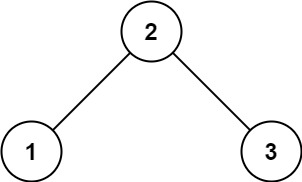# Leetcode 98 - Validate BST

Note:

• Use inorder traversal.
• If a tree is a BST, then its inorder traversal would be a strictly increasing array.

Given the root of a binary tree, determine if it is a valid binary search tree (BST).

A valid BST is defined as follows:

• The left subtree of a node contains only nodes with keys less than the node’s key.
• The right subtree of a node contains only nodes with keys greater than the node’s key.
• Both the left and right subtrees must also be binary search trees.

Example